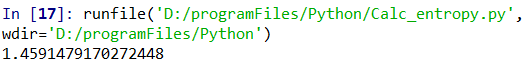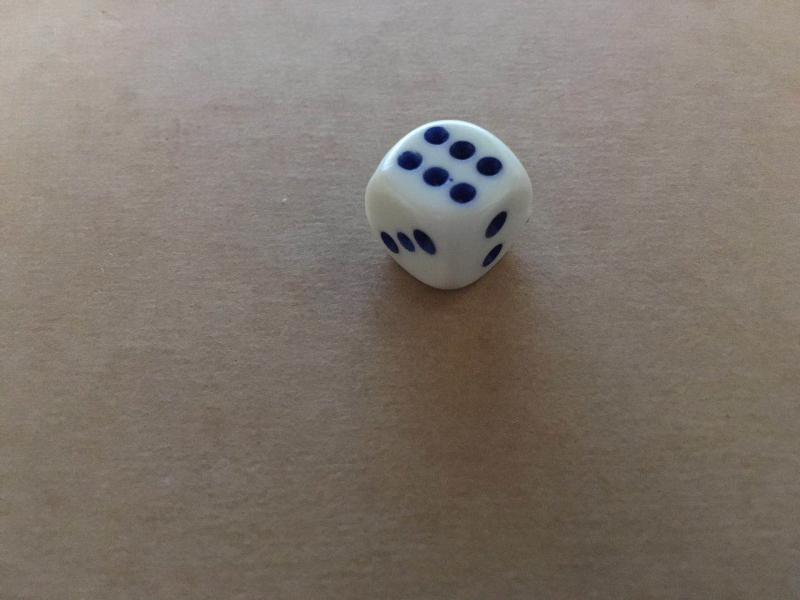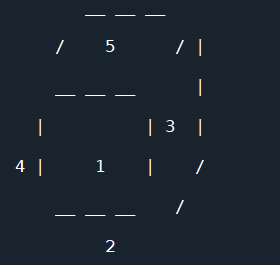• 提示：写完文章后，目录可以自动生成，如何生成可参考右边的帮助文档 文章目录系列文章目录古典概型与几何概型问题的Python解法1. 解古典概型问题2. 解2-维几何概型问题 古典概型与几何概型问题的Python解法 1. 解...
假定以$S$为样本空间的随机试验是一个等概模型，事件$A\subseteq S$。若能算得$|S|=n$，$|A|=m$，我们知道$P(A)=m/n$。我们把这样的计算方法定义成下列的Python函数。
from sympy import Rational  #导入Rational
def P(A, S):	            #等概模型下事件A的概率
n = len(S)	            #S所含元素个数
m = len(A)	            #A所含元素个数
return Rational(m, n)   #返回m/n的分数形式

结合程序中行内注释信息，不难理解上述程序。第3、4两行调用Python的len函数，分别计算由参数传递而来的S和A所含的元素个数n和m。第5行用m和n创建表示m/n的分数形式的Rational（sympy包中表示有理数的数据类型，第1行导入）对象并返回。
例1 将一枚均匀硬币抛掷三次，用上面定义的P函数计算事件$A_1$：“恰有一次出现正面”和$A_2$：“至少有1次出现正面”的概率$P(A_1)$和$P(A_2)$。
解： 若将分币的正面朝上记为1，正面朝下记为0，该随机试验的样本空间$S=\{(0, 0, 0), (0, 0, 1), (0, 1, 0), (0, 1, 1), (1, 0, 0), (1, 0, 1), (1, 1, 0), (1, 1, 1)\}$，即集合$\{0,1\}$中取3个元素可重排列的全体。故$|S|=8$。由于硬币是均匀的，所以每个样本点是等概的。事件“恰有一次出现正面”$A_1=\{(0, 0, 1), (0, 1, 0), (1, 0, 0)\}$，故$|A_1|=3$。
$P(A_1)= |A_1|/|S|=3/8$
事件“至少有1次出现正面” $A_2$的对立事件为“一次正面都没有出现”，即$\bar{A}_2=\{(0, 0, 0)\}$。故$|\bar{A}_2|=1$，于是$P(\bar{A}_2)=1/8$。而
$P(A_2)=1-P(\bar{A}_2)=1-1/8=7/8$
下列代码完成验算。
from sympy.utilities.iterables import variations as permutations#导入permutations
S=set(permutations([0, 1], 3, True))           					#构造样本空间S
A1 = setEvent(S, lambda a: sum(a) == 1)        					#设置事件A1
p1 = P(A1, S)                                  					#计算事件A1的概率p1
print('P(A1)=%s' % p1)                         					#输出p1
A2 = setEvent(S, lambda a: 1 in a)             					#设置事件A2
p2 = P(A2, S)                                  					#计算事件A2的概率p2
print('P(A2)=%s' % p2)                         					#输出p2

程序中第2行调用permutations函数（第1行导入，详见博文《排列组合——构造样本空间》）设置抛掷3次分币试验的样本空间S，其中的样本点为三元组(i, j, k)，每个分量均取1（正面朝上）或0（正面朝下）。第3行调用函数setEvent（定义见博文《按条件设置随机事件》），传递参数lambda a: sum(a)==1设置事件A1，第4行调用上面定义的计算等概模型中事件概率的函数P，用传递给它的参数A1和S计算A1的概率p1。第5行输出p1的值。相仿地，第8~10行分别设置事件A2，计算A2的概率p2，输出p2。运行程序，输出：
P(A1)=3/8
P(A2)=7/8

例2房间里有10个人，各自编号1~10，任选3人记录其编号。计算事件$A_1$：3人中最小编号为5，$A_2$：3人最大编号为5的概率$P(A_1)$和$P(A_2)$。
解： 在10个人中选择3人，共有$C_{10}^3$种不同的选法，即样本空间含有的样本点数$|S|= C_{10}^3=120$。又由于3人是任选的，所以每个选法发生的可能性是相同的。这是一个古典概型。事件$A_1$：所选3人中最小编号为5，意味着编号为5者必在此3人中。其余两人的编号应该比5大，共有$C_5^2=10$种选择方法，即$|A_1|=10$。于是
$P(A_1)= |A_1|/|S|=C_5^2/C_{10}^3=10/120=1/12$
相仿地，事件$A_2$：3人最大编号为5所含样本点数$|A_2|=C_4^2=6$。于是
$P(A_2)= |A_2|/|S|=C_4^2/C_{10}^3=6/120=1/20.$
下列代码完成验算
from sympy.utilities.iterables import subsets as combinations	#导入subsets
numbers=[1,2,3,4,5,6,7,8,9,10]                  				#房内10人的编号
S=set(combinations(numbers, 3))                 				#构造样本空间S
A1=setEvent(S, lambda a: min(a)==5)             				#3人中最小号码为5
p1=P(A1, S)
print('P(A1)=%s'%p1)
A2=setEvent(S, lambda a: max(a)==5)             				#3人中最大号码为5
p2=P(A2, S)
print('P(A2)=%s'%p2)

第2行将10个编号1,2,…,10设为列表numbers，第4行调用函数combinations（第1行导入，详见博文《排列组合——构造样本空间》），构造从numbers中任取3个的所有组合作为样本空间S。第4行函调用数setEvent设置事件$A_1$，最小号码为5；相仿地，第7行调用setEvent函数设置事件$A_2$，最大号码为5。运行程序，输出：
P(A1)=1/12
P(A2)=1/20

写博不易，敬请支持：
如果阅读本文于您有所获，敬请点赞、评论、收藏，谢谢大家的支持！


展开全文• 信息论的背后是：从小概率事件中学习到更多有价值的信息。而如何对信息进行度量最初起源于1948年香农提出的信息熵。它用于衡量变量的不确定性，变量的不确定性越大，熵也就越大。如热力学中的热熵表示分子状态的...
一、信息熵

信息论的背后是：从小概率事件中学习到更多有价值的信息。而如何对信息进行度量最初起源于1948年香农提出的信息熵。它用于衡量变量的不确定性，变量的不确定性越大，熵也就越大。如热力学中的热熵表示分子状态的混乱程度，熵越大，表示分子状态越混乱。

同时，信息熵在数学上可用信息的期望值表示。

假设对离散型事件 ，定义的信息为：

其中，表示变量在中出现的概率。

对事件求期望即为该事件的信息熵，记为

又对于离散随机变量的期望计算为：

则

其中，表示事件中元素分类的数量。

二、Python3.x实现信息熵的计算

假设有一组数据

1、首先计算出data的长度6，以及其中不重复元素为,和分类数量；

2、然后计算每个元素在data中出现的概率，分别为： ；

3、最后可计算出该数据的信息熵为：

其Python实现代码如下：

import numpy as np

data=['a','b','c','a','a','b']
data1=np.array(data)#将list转换为array，使用属性shape，用于统计元素个数

#计算信息熵的方法
def calc_ent(x):
"""
calculate shanno ent of x
"""
#x.shape计算数组x的元素长度，x长度为x.shape=6
#set() 函数创建一个无序不重复元素集
x_value_list = set([x[i] for i in range(x.shape)])#得到数组x的元素（不包含重复元素），即x_value_list={'c', 'b', 'a'}
#    print(x_value_list)
ent = 0.0
for x_value in x_value_list:
p = float(x[x == x_value].shape) / x.shape#计算每个元素出现的概率
#        print(p)
logp = np.log2(p)
ent -= p * logp
print(ent)

if __name__ == '__main__':
calc_ent(data1)

其输出结果如图：即该data的信息熵为1.4591479170272448。

展开全文• 掷色子的问题总会出现在概率课本中，老师们都喜欢拿这个耳熟能详的事件来举例子，但今天我们不讲概率，我们来用Python模拟掷色子。 用random模块我们可以轻易地模拟输出点数（一般我们取的这个点数是色子顶上的点数...
掷色子的问题总会出现在概率课本中，老师们都喜欢拿这个耳熟能详的事件来举例子，但今天我们不讲概率，我们来用Python模拟掷色子。
用random模块我们可以轻易地模拟输出点数（一般我们取的这个点数是色子顶上的点数），即随机生成一个1-6的数字。
random.randint(1,6)

的确非常容易，但如果我们想要知道色子的正面反面，左面右面的数值，又该如何计算呢？
本着求知探索精神， 我翻箱倒柜找到一枚祖传色子，来仔细观察一下。我们可以发现，每个点数的对面点数相加都为7，即1的对面点数为6，剩下的2，3，4，5也对称的分布在1的四侧（2，5相对，3，4相对）。但是其四面的顺序，从不同的角度观测其排列顺序方式也是不同的，还是以顶上点数1为例，从点数2开始按照顺时针我们可以看到2，4，5，3，从点数4开始按照顺时针我们可以看到4，5，3，2，而这些序列构成一个环状。而这些环状数组，我们可以直接用列表来表示，1-6点的顺时针侧面点数列表如下所示
[2,4,5,3]
[1,3,6,4]
[1,5,6,2]
[1,2,6,5]
[1,4,6,3]
[2,3,5,4]

因此，想要描述一个色子的每一面就有了思路，首先随机生成一个数字，直接当作顶部的数字；接着随机生成一个状态，表示从其对应数组的某一位开始，也可以直观地理解为从不同角度看过去；最后输出不同位置的点数，用手绘立方体的方式打印出色子。
rand_number=random.randint(0,5)
rand_state=random.randint(0,3)

def throw():
print("         __ __ __ \n");
print("      /    %d      / |\n"%(rand_number+1))
print("      __ __ __      |\n")
print("    |          | %d  |\n"%dice[rand_number][(rand_state+1)%4])
print("  %d |     %d    |    /\n"%(dice[rand_number][(rand_state+3)%4],dice[rand_number][(rand_state+2)%4]))
print("      __ __ __    /\n")
print("           %d\n"%(6-rand_number))

下面请欣赏我的灵魂画作之画色子~展开全文• 【知识铺垫】在介绍如何使用贝叶斯概率公式计算后验概率之前，先回顾一下概率论与数理统计中的条件概率和全概率公式：如上等式为条件概率计算公式，表示在已知事件A的情况下事件B...


【知识铺垫】在介绍如何使用贝叶斯概率公式计算后验概率之前，先回顾一下概率论与数理统计中的条件概率和全概率公式：如上等式为条件概率的计算公式，表示在已知事件A的情况下事件B发生的概率，其中P(AB)表示事件A与事件B同时发生的概率。所以，根据条件概率公式得到概率的乘法公式：。事件A的概率可以根据全概率公式计算得到：如上等式即为全概率公式，其中事件构成了一个完备的事件组，并且每一个均大于0。该公式表示，对于任意的一个事件A来说，都可以表示成n个完备事件组与其乘积的和。【贝叶斯公式】在具备上述的基础知识之后，再来看看贝叶斯公式。如前文所说，贝叶斯分类器的核心就是在已知X的情况下，计算样本属于某个类别的概率，故这个条件概率的计算可以表示为：其中，表示样本所属的某个类别。假设数据集的因变量y一共包含k个不同的类别，故根据全概率公式，可以将上式中的分母表示成；再根据概率的乘法公式，可以将上式中的分子重新改写为。对于上面的条件概率公式而言，样本最终属于哪个类别，应该将计算所得的最大概率值对应的类别作为样本的最终分类，所以上式可以表示为：如上公式所示，对于已知的X，朴素贝叶斯分类器就是计算样本在各分类中的最大概率值。接下来详细拆解公式中的每一个部分，为获得条件概率的最大值，寻找最终的影响因素。分母是一个常量，它与样本属于哪个类别没有直接关系，所以计算的最大值就转换成了计算分子的最大值，即；如果分子中的项未知的话，一般会假设每个类别出现的概率相等，只需计算的最大值，然而在绝大多数情况下，是已知的，它以训练数据集中类别的频率作为先验概率，可以表示为。所以，现在的主要任务就是计算的值，即已知某个类别的情况下自变量为某种值的概率。假设数据集一共包含p个自变量，则可以表示成，进而条件概率可以表示为：很显然，条件联合概率值的计算还是比较复杂的，尤其是当数据集的自变量个数非常多的时候。为了使分类器在计算过程中提高速度，提出了一个假设前提，即自变量是条件独立的（自变量之间不存在相关性），所以上面的计算公式可以重新改写为：如上式所示，将条件联合概率转换成各条件概率的乘积，进而可以大大降低概率值的运算时长。但问题是，在很多实际项目的数据集中，很难保证自变量之间满足独立的假设条件。根据这条假设，可以得到一般性的结论，即自变量之间的独立性越强，贝叶斯分类器的效果就会越好；如果自变量之间存在相关性，就会在一定程度提高贝叶斯分类器的错误率，但通常情况下，贝叶斯分类器的效果不会低于决策树。自变量X的数据类型可以是连续的数值型，也可以是离散的字符型，或者是仅含有0-1两种值的二元类型。通常会根据不同的数据类型选择不同的贝叶斯分类器，例如高斯贝叶斯分类器、多项式贝叶斯分类器和伯努利贝叶斯分类器。【高斯贝叶斯分类器】如果数据集中的自变量X均为连续的数值型，则在计算时会假设自变量服从高斯正态分布，所以自变量的条件概率可以表示成：其中，表示第j个自变量的取值，为训练数据集中自变量属于类别的均值，为训练数据集中自变量属于类别的标准差。所以在已知均值和标准差时，就可以利用如上的公式计算自变量取某种值的概率。【多项式贝叶斯分类器】如果数据集中的自变量X均为离散型变量，就无法使用高斯贝叶斯分类器，而应该选择多项式贝叶斯分类器。在计算概率值时，会假设自变量X的条件概率满足多项式分布，故概率值的计算公式可以表示为：其中，表示自变量的取值；表示因变量为类别时自变量取的样本个数；表示数据集中类别的样本个数；为平滑系数，用于防止概率值取0可能，通常将该值取为1，表示对概率值做拉普拉斯平滑；n表示因变量的类别个数。 【伯努利贝叶斯分类器】当数据集中的自变量X均为0-1二元值时（例如在文本挖掘中，判断某个词语是否出现在句子中，出现用1表示，不出现用0表示），通常会优先选择伯努利贝叶斯分类器。利用该分类器计算概率值时，会假设自变量X的条件概率满足伯努利分布，故概率值的计算公式可以表示为：其中，为第j个自变量，取值为0或1；表示类别为时自变量取1的概率，该概率值可以使用经验频率代替，即其中，表示类别的样本个数；表示在类别为时，变量取1的样本量；为平滑系数，同样是为了避免概率为0而设置的；n为因变量中的类别个数。有关贝叶斯算法的原理就介绍到这里，除此，如何借助于简单的案例解释原理背后的道理，可以在我的新书《从零开始学Python数据分析与挖掘》中得到详细的答案。【结语】OK，关于贝叶斯算法的理论知识我们就分享到这里，如果你有任何问题，欢迎在公众号的留言区域表达你的疑问，下一期我们将分享三种贝叶斯算法的实战案例。同时，也欢迎各位朋友继续转发与分享文中的内容，让更多的人学习和进步。


展开全文• python字符串与绘图复习变量None变量参与运算没有赋值的情况不会改变绘制立方体绘制五角星时间计算强化实现贷款计算连续输入多个字符串加法与绘图实现显示线段长度数据函数使用快速查阅函数帮助字符串三种风格字符...
• 这些是作为计算离散分布之间的距离和散度的快速解决方案，尤其是当两个分布包含大量概率为零的事件（在词典中未描述）时。 如何安装此软件包？ 和往常一样，只需使用pip下载即可： pip install dictances 测试...# python如何计算概率事件python 订阅